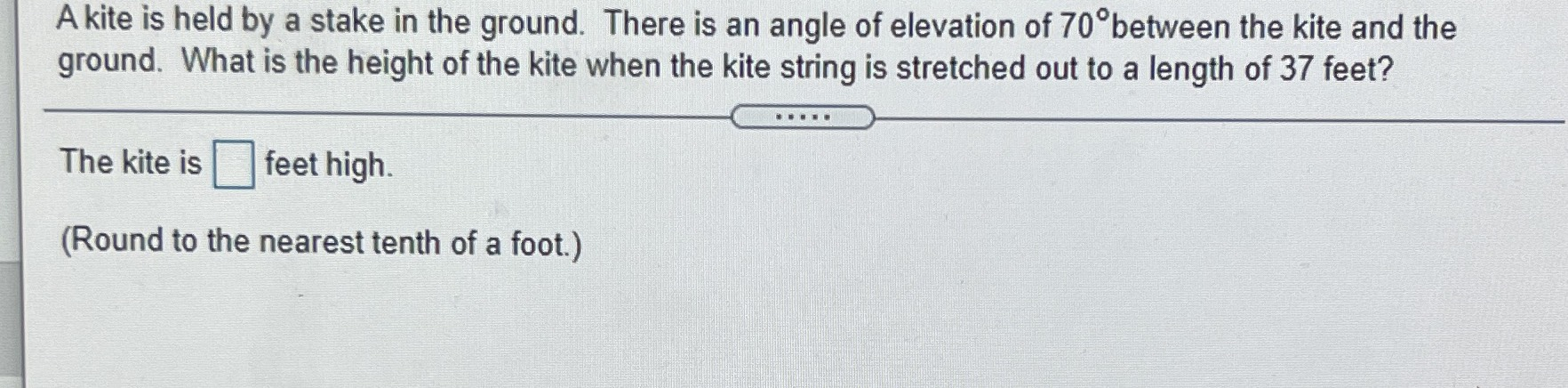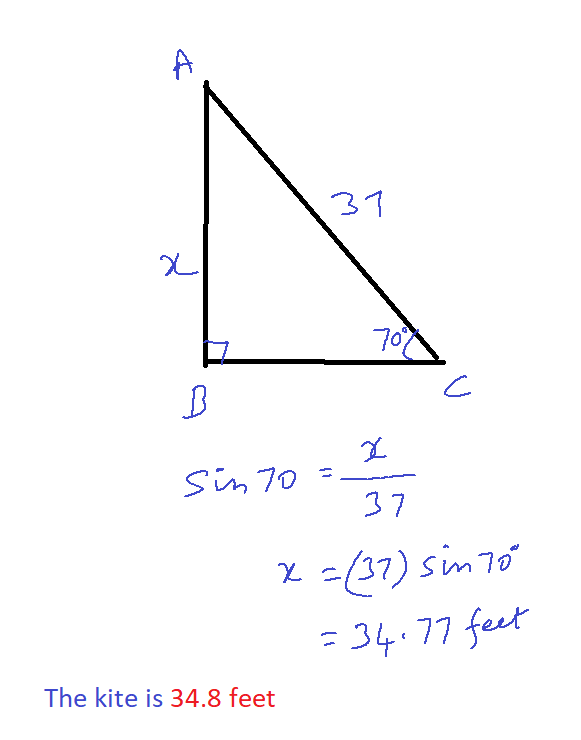### ¿Todavía tienes preguntas de matemáticas?

Pregunte a nuestros tutores expertos
Algebra
PreguntaA kite is held by a stake in the ground. There is an angle of elevation of $$70 ^ { \circ }$$ between the kite and the ground. What is the height of the kite when the kite string is stretched out to a length of $$37$$ feet?

The kite is $$\square$$ feet high. (Round to the nearest tenth of a foot.)